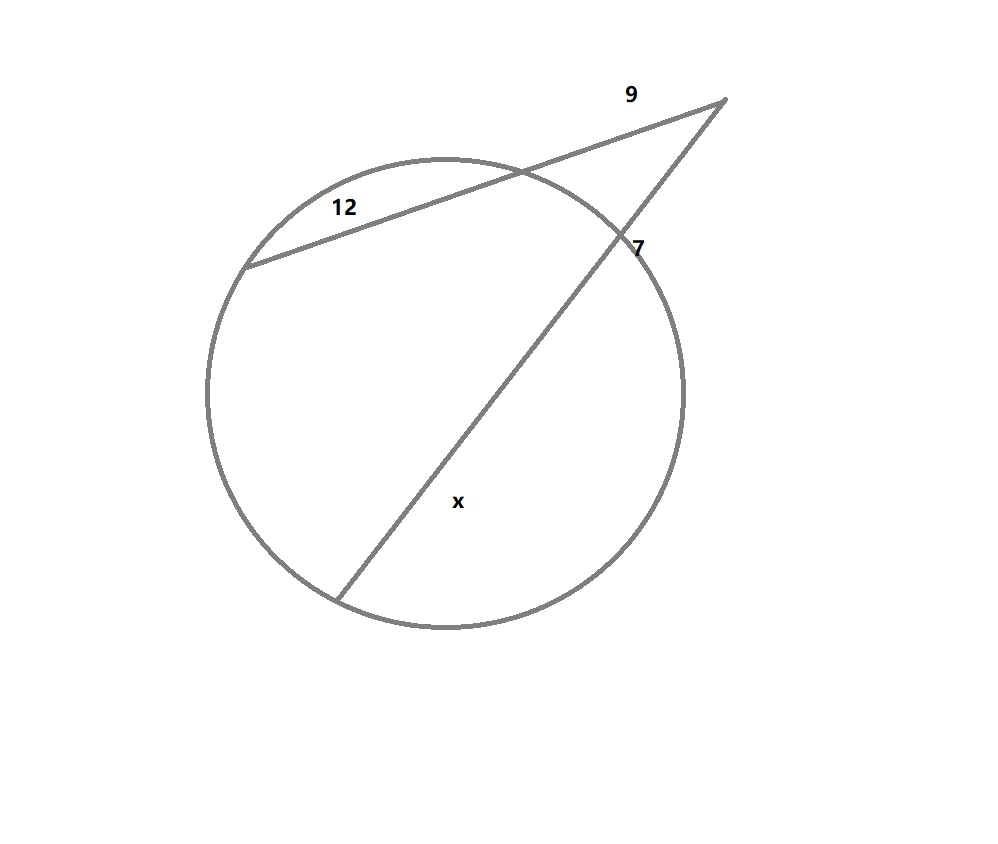Solve for x FSP19611001241.jpgFSZFrancisca Rodden 2021-12-18 Answered
Solve for x• Questions are typically answered in as fast as 30 minutes

Solve your problem for the price of one coffee

• Math expert for every subject
• Pay only if we can solve itMaricela Alarcon
Step 1
We have to use analytical geometry property here.
By analytic Geometry
$$\displaystyle\Rightarrow{9}{\left({9}+{12}\right)}={7}{\left({7}+{x}\right)}$$
$$\displaystyle\Rightarrow{189}={49}+{7}{x}$$
$$\displaystyle\Rightarrow{140}={7}{x}$$
$$\displaystyle{x}={\frac{{{140}}}{{{7}}}}={20}$$
Answer: $$\displaystyle={20}$$
Not exactly what you’re looking for?trisanualb6
Step 1
Factor out x on the left side.
$$\displaystyle{\left({c}-{b}\right)}{x}={a}$$
Divide $$\displaystyle{c}-{b}$$ to both sides
$$\displaystyle{\frac{{{\left({c}-{b}\right)}{x}}}{{{c}-{b}}}}={\frac{{{a}}}{{{c}-{b}}}}$$
$$\displaystyle{x}={\frac{{{a}}}{{{c}-{b}}}}$$nick1337

Step 1
Given equation:
$$9(9+12)=7(7+x)$$
Rewrite the equation as:
$$7(7+x)=9(9+12)$$
Simplify.
$$7(7+x)=9\times21$$
Multiply 9 by 21
$$7(7+x)=189$$
Divide each term in
$$7(7+x)=189$$
by 7 and simplify
$$\frac{7(7+x)}{7}=\frac{189}{7}$$
Simplify the left side.
Cancel the common factor.
$$\frac{{7}(7+x)}{{7}}\frac{189}{7}$$
Divide 7+x by 1
$$7+x=\frac{189}{7}$$
Simplify the right side.
Divide 189 by 7

7+x=27
Move all terms not containing x to the right side of the equation.
Subtract 7 from both sides of the equation.
x=27-7
Subtract 7 from 27
x=20